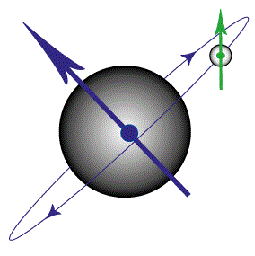AIR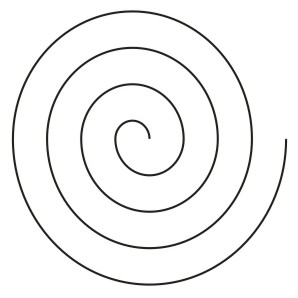Spiral Symbol of Air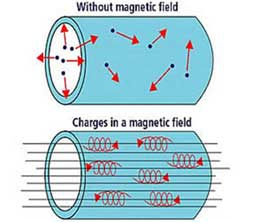Magnet Field and Electrical Charges

In a magnetic field, any moving particles are forced to spiral along the magnetic field lines, because they are charged. So, if you create a circular magnetic field, then plasma particles will be trapped, perpetually circulating around the loop.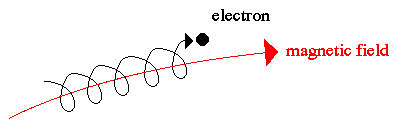Electron's Spiral

In a magnetic field, any moving particles are forced to spiral along the magnetic field lines, because they are charged. So, if you create a circular magnetic field, then plasma particles will be trapped, perpetually circulating around the loop.

If an electron’s motion closely parallels the magnetic lines of force it will still be deflected somewhat by the magnetic field, but now it will be forced into a spiral that follows the magnetic lines of force out of the magrid, and around one of the coil cross-sections. Electrons that spiral along the magnetic lines of force out of the magrid and back in again, are said to be circulating.

If the electron and the proton (or other positive ion) were free, they would simply move in those directions. But they are not free, because of the magnetic force.

If only magnetic forces were present (no electric field), the proton would circle around a magnetic field line in the clockwise direction (from where we are looking) and the electron in the counter-clockwise direction.Electrons Spiral Counter Clockwise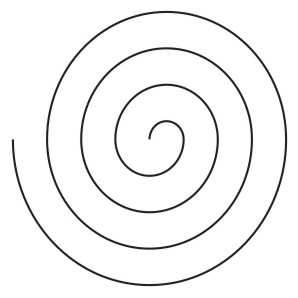Protons Spiral Clockwise

Why electric fields parallel to magnetic field lines are rare in space :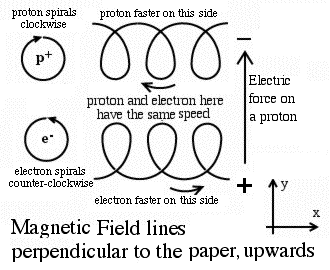It will be assumed in what follows that the direction of the electric force ("direction of the electric field") is always perpendicular to the local direction of magnetic field lines. Particle drifts in electric + magnetic fields

There exists a reason. In space, ions and electrons spiral around their guiding magnetic field lines, but at the same time they can also slide along those lines, like beads threaded on a wire.

If the electric force had some part in that direction (a "vector component"), those ions and electrons, as they advance along their guiding field lines, would also be accelerated by it, and gain speed. However gaining speed also means gaining energy. Because energy in nature is conserved, whatever the particle gains, weakens the accelerating part of the electric field, and unless fresh energy is constantly supplied, that part does not last long.

Without such fresh energy (the usual case), the electric force along the field line quickly drops to zero. When that happens, the same voltage exists at all points along a magnetic field line, leaving no voltage differences that might drive currents in that direction. The remaining electric field is then perpendicular to the magnetic field lines.

There energy is being supplied and the electric force does have a component in the same direction as the magnetic field line.

The Electric Force :The local magnetic field lines in the drawing (repeated here for convenience) are assumed to be perpendicular to the paper, coming out towards you. Suppose also the electric field representing the electric force is in the plane of the drawing, towards the top of the figure. A straight arrow was drawn giving that direction, which we choose to be the y direction in a system of (x, y) axes, drawn in the bottom right corner.

A positive particle such as a proton, marked here p+ is pushed by the electric force towards the top of the drawing, in the +y direction.

A negative electron, marked e-, is pushed towards the bottom, in the -y direction You can imagine a positive charge and a negative charge somewhere above it, creating that force repelling or attracting the proton or electron.

The Magnetic Force Alone :

If the electron and the proton (or other positive ion) were free, they would simply move in those directions. But they are not free, because of the magnetic force.

If only magnetic forces were present (no electric field), the proton would circle around a magnetic field line in the clockwise direction (from where we are looking) and the electron in the counter-clockwise direction.

Electric and Magnetic Forces together :

The electric force modifies the motion. Protons are accelerated in the +y direction, so they move a bit faster on the part of their circle.

Electrons are accelerated in the -y direction, so their speed is a bit greater on the part of their circle closer to the bottom.

Faster ions or electrons circle with a bigger radius. They behave a bit like a racing car: the greater its speed, the wider is the circle it follows when going around a curve.

Therefore protons make wider circles at the top of their circles, and electrons make wider circles at the bottom of their circles. The result is a slow crablike sideways motion ("drift") in the (-y) direction, by both ions and electrons. Even though they circle their field lines in opposite directions, the electric field moves them both in the same direction, to the right.

It can also be shown that the velocity of both motions is always the same even though protons are nearly 2000 times heavier, and even though the initial energies of the particles can be very different. (To those familiar with mathematics and physics, this process can be explained much more concisely and transparently.) The result is always a sideways flow of the plasma, a migration of the entire plasma, a bulk motion of the gas rather than a flow of electric current.

Magnetic Drifts :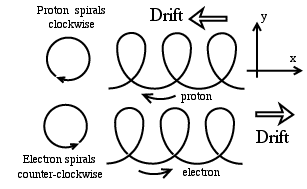Electric currents without any voltage :

Suppose as before that magnetic field lines are perpendicular to the drawing, and that the same (x, y) axes are used as before. Only now no electric field exists, and instead the strength of the magnetic force changes with distance in the y direction it is much greater at the top of the drawing than at the bottom.

As before both ions and electrons circle around magnetic field lines. However, the size of the circle also depends on the strength of the magnetic force the stronger the force, the smaller the radius of the circle. (In the limit where the magnetic force drops to zero, the particles move in straight lines same as circles of infinite radius)

Because the way the strength of the force changes, the orbits, again, are no longer circles but flat spirals, curving more sharply at the top of their motion.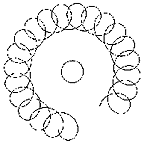The result as before, is again a crab like sideways "drift." This time, however, protons and electrons drift in opposite directions. Protons move to the left, electrons to the right, and both motions contribute a right-to-left electric current.

All field lines point upwards and the strength of the magnetic field increases inwards, towards the Earth. The drift is therefore in the 3rd perpendicular direction, which carries the particles around Earth electrons counter clockwise, protons clockwise, and the current flows clockwise too.